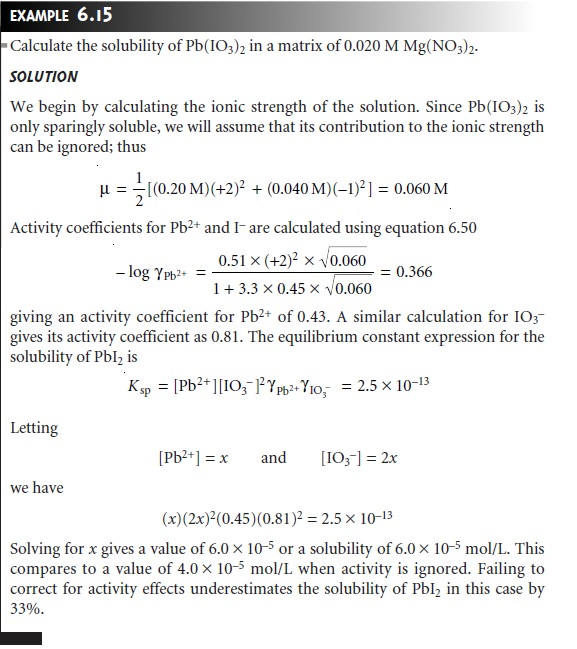Home | | Modern Analytical Chemistry | Activity Effects

# Activity Effects

Suppose you need to prepare a buffer with a pH of 9.36.

Activity Effects

Suppose you need to prepare a buffer with a pH of 9.36. Using the Hendersonâ€“ Hasselbalch equation, you calculate the amounts of acetic acid and sodium acetate needed and prepare the buffer. When you measure the pH, however, you find that it is 9.25. If you have been careful in your calculations and measurements, what can account for the difference between the obtained and expected pHs? In this section, we will examine an important limitation to our use of equilibrium constants and learn how this limitation can be corrected.

Careful measurements of the solubility of AgIO3 show that it increases in the presence of KNO3, even though neither K+ or NO3â€“ participates in the solubility reaction. Clearly the equilibrium position for the reaction

AgIO3(s)  < == === >  Ag+(aq)+ IO3â€“(aq)

depends on the composition of the solution. When the solubility product for AgIO3 is calculated using the equilibrium concentrations of Ag+ and IO3â€“

Ksp = [Ag+][IO3â€“]

its apparent value increases when an inert electrolyte such as KNO3 is added.

Why should adding an inert electrolyte affect the equilibrium position of a chemical reaction? We can explain the effect of KNO3 on the solubility of AgIO3 by considering the reaction on a microscopic scale. The solution in which equilibrium is established contains a variety of cations and anionsâ€”K+, Ag+, H3O+, NO3â€“, IO3â€“ and OHâ€“. Although the solution is homogeneous, on the average, there are more anions in regions near Ag+ ions, and more cations in regions near IO3â€“ ions. Thus, Ag+ and IO3â€“ are surrounded by charged ionic atmospheres that partially screen the ions from each other. The formation of AgIO3 requires the disruption of the ionic atmospheres surrounding the Ag+ and IO3â€“ ions. Increasing the concentrations of ions in solution, by adding KNO3, increases the size of these ionic atmospheres. Since more energy is now required to disrupt the ionic atmospheres, there is a decrease in the formation of AgIO3, and an apparent increase in the equilibrium constant.

The ionic composition of a solution frequently is expressed by its ionic strength, ÎĽwhere ci and zi are the concentration and charge of the ith ion.Note that the unit for ionic strength is molarity, but that the molar ionic strength need not match the molar concentration of the electrolyte. For a 1:1 electrolyte, such as NaCl, ionic strength and molar concentration are identical. The ionic strength of a 2:1 electrolyte, such as Na2SO4, is three times larger than the elec- trolyteâ€™s molar concentration.

The true thermodynamic equilibrium constant is a function of activity rather than concentration. The activity of a species, aA, is defined as the prod- uct of its molar concentration, [A], and a solution-dependent activity coeffi- cient, ÎłA.The true thermodynamic equilibrium constant, Ksp, for the solubility of AgIO3, therefore, is

Ksp = (aAg+)(aIO3 â€“) = [Ag+][IO3-](ÎłAg+)(ÎłIO3â€“)

To accurately calculate the solubility of AgIO3, we must know the activity coeffi- cients for Ag+ and IO3 â€“.

For gases, pure solids, pure liquids, and nonionic solutes, activity coefficients are approximately unity under most reasonable experimental conditions. For reac- tions involving only these species, differences between activity and concentration are negligible. Activity coefficients for ionic solutes, however, depend on the ionic composition of the solution. It is possible, using the extended Debyeâ€“HĂĽckel the- ory,* to calculate activity coefficients using equation 6.50where ZA is the charge of the ion, Î±A is the effective diameter of the hydrated ion in nanometers (Table 6.1), ÎĽ is the solutionâ€™s ionic strength, and 0.51 and 3.3 are con- stants appropriate for aqueous solutions at 25 Â°C.Several features of equation 6.50 deserve mention. First, as the ionic strength approaches zero, the activity coefficient approaches a value of one. Thus, in a solu- tion where the ionic strength is zero, an ionâ€™s activity and concentration are identi- cal. We can take advantage of this fact to determine a reactionâ€™s thermodynamic equilibrium constant. The equilibrium constant based on concentrations is mea- sured for several increasingly smaller ionic strengths and the results extrapolated back to zero ionic strength to give the thermodynamic equilibrium constant.

Second, activity coefficients are smaller, and thus activity effects are more important, for ions with higher charges and smaller effective diameters. Finally, the extended Debyeâ€“HĂĽckel equation provides reasonable activity coefficients for ionic strengths of less than 0.1. Modifications to the extended Debyeâ€“HĂĽckel equation, which extend the calculation of activity coefficients to higher ionic strength, have been proposed.As this example shows, failing to correct for the effect of ionic strength can lead to significant differences between calculated and actual concentrations. Neverthe- less, it is not unusual to ignore activities and assume that the equilibrium constant is expressed in terms of concentrations. There is a practical reason for thisâ€”in an analysis one rarely knows the composition, much less the ionic strength of a sample solution. Equilibrium calculations are often used as a guide when developing an an- alytical method. Only by conducting the analysis and evaluating the results can we judge whether our theory matches reality.

Study Material, Lecturing Notes, Assignment, Reference, Wiki description explanation, brief detail
Modern Analytical Chemistry: Equilibrium Chemistry : Activity Effects |